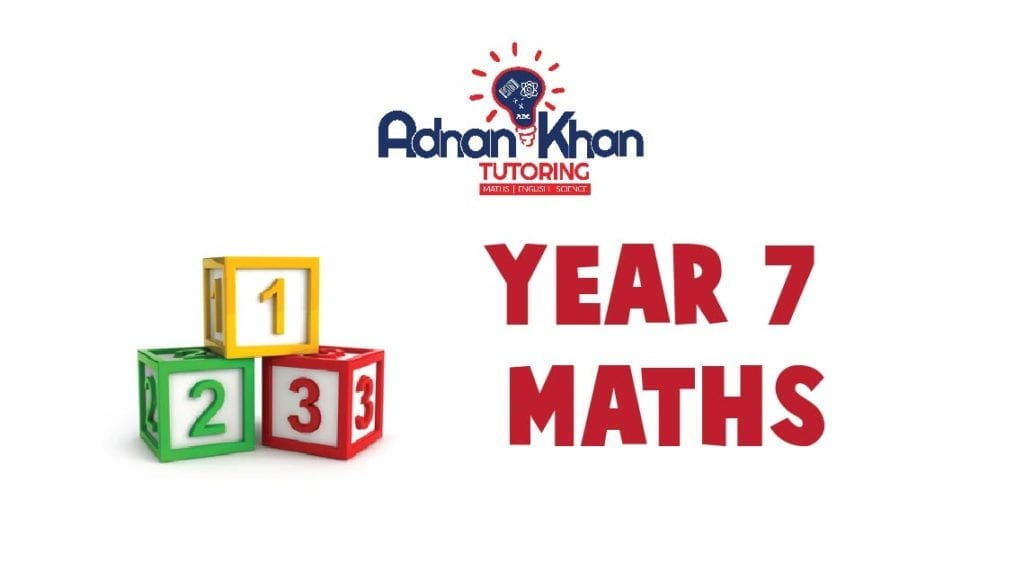# Year 7 Maths Tutors in High Wycombe

Year 7 is the start of secondary education. Students take 11 plus exams to get into secondary schools. It is also the start of key stage 3, and the subjects get more challenging. Math is the most demanding subject, and the year 7 curriculum needs a lot of practice. Parents hire year 7 Maths tutors in High Wycombe to get the child exclusive Maths tuition. Adnan Khan Tutoring understands the learning needs of the child. Some parents prefer private tutor for year 7 in High Wycombe to provide the child with a one-to-one learning environment.

We follow the UK’s national curriculum guidelines in providing our tuition services. We have more than 20 years of experience in helping the students. Our year 7 Maths tuition is unique that follows the new learning and tutoring methods. We are a well-reputed tutoring agency in High Wycombe. Our expert online tutors take regular live classes and provide the best year 7 Maths tutoring in High Wycombe and the UK. We keep the parents in the loop by providing regular feedback and weekly reports.

## What lessons do we cover in our online year 7 maths tuition?

Our online year 7 Maths lessons are divided into four sections. The first section is Number Crunching which includes lessons based on different calculations.

• The second section is Shapes and includes the lessons, Solids and Nets, Volume and Capacity, Symmetry, Shapes You Need To Know, Regular Polygons, Rounding Off, Estimating and Conversion Graphs.
• The third section of the year 7 Maths course is Shape, Space and Measures with lessons: Length, perimeter and area, Perimeter and area of rectangles, 3-D shapes, Surface area of cubes and cuboids, Lines and angles and more.
• The fourth section includes the lesson under Handling Data. The topics include Mode, median and range Using a tally chart, Using the correct data, Grouped frequencies, Data collection, Pie charts, Comparing data and Statistical surveys.

Year 7 MATHS Topics

Algebra

• Sequences and rules
• Finding missing terms
• Finding the general term (nth term)
• Functions and mappings
• Using letter symbols
• Algebraic terms and expressions
• Rules of algebra
• Simplifying expressions
• Formulae
• Equations
• Square numbers and square roots
• Triangle numbers
• From mappings to graphs
• Square-and-circle problems
• Solving equations
• Graphs from the real world

Number

• Decimals
• Ordering decimals
• Directed numbers
• Estimates
• Fractions
• Fractions and decimals
• Multiplying and dividing decimals
• Equivalences
• Rounding
• The four operations
• BODMAS
• Long multiplication and division
• Calculating with measurements
• Ratio and proportion
• Calculating ratios and proportions
• Using a calculator

Shape, Space and Measures

• Length, perimeter and area.
• Perimeter and area of rectangles.
• 3-D shapes.
• Surface area of cubes and cuboids
• Lines and angles
• Coordinates
• Measuring and drawing angles
• Constructions
• Solving geometrical problems
• Line symmetry
• Rotational symmetry
• Reflections
• Translation
• Polygons

Handling Data

• Mode, median and range
• The mean
• Statistical diagrams
• Probability
• Experimental probability
• Using a tally chart
• Using the correct data
• Grouped frequencies
• Data collection
• Pie charts
• Comparing data
• Statistical surveys

## Enrol Now

#### £129.00

per month for 12 total payments
12 months of access

Categories:

Scroll to Top